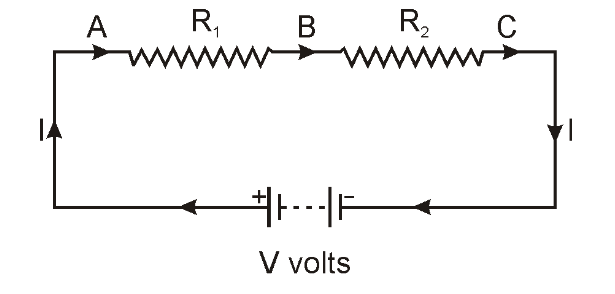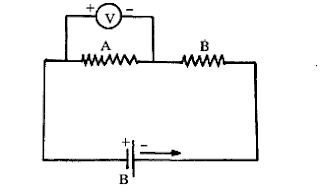# CBSE Class 10 - Electricity - Questions and Answers

Q1: What is the charge on an electron?

Q2: How much electron are required for 1 Coulomb charge?

Q3: What is the SI unit of charge?

Q4: Which type of circuitis requid for electricity?

Q5: Which instrument is used for measuring the current in electric circuit?

Q6: What is the conventinal direction of current in a circuit?

Answer: Opposite to the direction of flow of electron

Q7: What is the SI unit of mesurement of current?

Q8: What is the meaning of 1A current in a conductor?

Answer: 1A current in a conductor means 1 coulomb of charge passing any cross-sectional area of conductor in 1 sec.

Q9: What do you mean by electric current?

Answer: Electric current means flow of charge or electron inside the conducting wire.

Q10: What is the CGS unit of charge?

Q11: Write the unit of electric potensial.

Answer: Volt (V) or Joule per Coulomb

Q12: Define the potensial at a point.

Answer: Potensial of a point is equal to workdone to bring a change of one coulomb from infinity to the point.

Q13: Define the potensial difference between two points.

Answer: Potenisal difference of two points is equal to workdone to bring a charge of one coulomb from one point to another.

Q14: A dry cell usually has a small cap at one end and a flat surface at the other end. Which of the two is at a higher potenisal?

Answer: Small cap of dry cell represents positive terminal of the cell, and flat surface of dry cell represents negative terminal of the cell. So, the small cap (positive terminal) have more potenisal then flat surface (negative terminal).

Q15: Name the instrument used to measure eletric current and potensial difference respectively. Which of these is connented in series and which is connented in parallel in a circuit?

Answer: The instrument used to measure eletric current and potensial difference are ammeter and voltmeter respectively. Ammeter is always connected in series, whereas Voltmeter is always connected in parallel in a circuit.

Q16: What is the shape of graph between V and i, where V is the potenisal difference between the ends of a wire and i is the current in it.

Answer: The shape of graph between V and i is always straight and its slop tells about resistance of the wire.

Q17: Consider the units volt, ohm and ampere. One of them is the same as the product of the other two. Which one is this?

Answer: Volt is the product of ohm and ampere.

Q18: Name three electrical appliance in which the heating effect of electric current is used.

Answer: Heater, Electric iron, Induction plate

Q19: You have two resistors of resistance of 30Ω and 60Ω. Which resistance can you get by combining the two?

Answer: If they combined in series, their equivalent resistance is 90Ω.
Whereas, if they combined in parallel, their equivalent resistance is 10Ω.

Q20: Draw a diagram to show two resistors R1 and R2 connected in series.Q21: Two resistors of 5Ω and 10Ω are connected in series in a circuit. How does the current passing through them compare?

Answer: Both the resistors 5Ω and 10Ω are connected in series in a circuit. So, there is no change in the current passing through them.

Q22: A wire of resistance of 10Ω is bent to form a closed circle. What is the resistance across a diameter of the circle.

Answer: The resistance across a diameter of the circle is 2.5Ω.

Q23: What is the difference between conductor and insulator? Give one example of each.

Answer: Conductor:- It has very low resistivity. Such as: Silver
Insulators:- It has very high resistivity. Such as: Wood

Q24: A wire of resistance of 10Ω is bent to form a closed square. What is the resistance across the opposite cornors of the square.

Answer: The resistance across the opposite cornors of the square is 2.5Ω.

Q25: The current in a wire is one ampere. Explain this statement in terms of the charge flowing through the wire.

Answer: When one coulomb of charge passing through any cross-section of the wire in one second, then we say one ampere current is passing through the wire.

Q26: The current in a wire is one ampere. Explain this statement in terms of number of electrons flowing through the wire.

Answer: When 6.25 x 10^18 electrons passing through any cross-section of the wire in one second, then we say one ampere current is passing through the wire.

Q27: When do you say that the resistance of a wire is 1Ω?

Answer: When a potential difference of 1 volt (1V) applied across a wire produce a current of 1 ampere (1A), then we say resistence of wire is 1 ohm (1Ω).

Q28: Draw a circuit diagram for a circuit in which two resistors A and B are connected in series with a battery and a voltmeter is connected to measure the potential difference across the resistor A.Q29: When are resistor is said to be connected in a series.

Answer: When two or more resistors are connected end to end. Then they are said to be in series combination of resistors.

Q30: When are resistor is said to be connected in a parallel.

Answer: When two or more resistors are connected between two common end points. Then they are said to be in parallel combination of resistors.

Q31: Why is tungesten not used as a fuse wire?

Answer: Tungesten is not used as a fuse wire because it has a very high melting point which will not melt when high current will pass through the circuit and the fuse will not work properly.

Q32: Why is tungesten suitable for making the filament of a bulb?

Answer: Tungesten is suitable for making the filament of a bulb because it has very high resistivity, which creates more amount of heat and emits light.

Q33: Silver is a better conductor of electricity than copper. Why then do we use copper wire for conducting electricity?

Answer: We use copper wire for conducting electricity insted of silver because copper is much cheaper material and it is more ductile than silver. Fine wire of copper can be made with least resistance which is not possible in silver.

Q34: What do you mean by heating effect of current?

Answer: When current is passed through a conductor, it works against the resistance of the conducting wire. This work is converted into heat energy, which heat up the conducting wire. This phenomenon is known as heating effect of the current.

Q35: Power of a lamp is 60W. Find the energy in joules consumed by it in 1 sec.

Answer: 60W means 60J/s, so energy consumed is 60J.

Q36: Why do we use copper and aluminium wire for transmission of electric current?

Answer: Copper and Aluminium wires are used for transmission of electric current because they have very low resistance.

Q37: Give one example of a metal which is best conductor of heat?

Q38: Nichrome is used to make element of electric heater. Why?

Answer: Nichrome is used to make element of electric heater because it is an alloys which has a high resistance and high melting.

Q39: What happens to the resistance of a conductor when temperature is incresed?

Answer: The resistance of a conductor will increase when temperature is incresed.

Q40: Why is tungsten metal selected for making filaments of incandescent lamp?

Answer: Tungsten metal is selected for making filaments of incandescent lamp because it has a high resistance and high melting point.

Q41: Write down the relation between heat produced H in a conductor of resistance R through which current I passes for t seconds.

Q42: State Joule’s law of heating.

Answer: The Joule's laws say that the amount of heat produced in a conductor is directly proportional to the square of electric current passing through the conductor, directly proportional to the resistance of the conductor, and directly proportional to the time for which electric current passes through the conductor.

Q43: What is the formula for Joule’s law of heating?

Q44: What is meant by the statement that the rating of fuse in a circuit is 5A?

Answer: This statement means that the maximun current of 5A can flow from the circuit.

Q45: Define electric power.

Answer: Electric power means the amount of electric energy consumed in an electric circuit per unit time.

Q46: Name the physical quantity expressed as the product of potential difference and electric current.

Q47: State SI unit of electric power.

Q48: What is the commercial unit of energy ?